# Quiz 5: Costing for Nonroutine Decisions

Business

Sunk cost refers to those costs that have already been incurred and thus cannot be recovered. Nonroutine decisions are the decisions which are taken when an uncommon situation occurs. These are those situations which the manager has never dealt before and not even similar ones. So, Nonroutine decision making is quite risky and includes lot of anxiety and stress. Sunk cost should be carefully excluded from the Nonroutine decision analysis. This is because the key in non routine analysis is to look to the future rather than past but sunk cost is the costs that has already incurred in the past. This is the reason why sunk costs should be not be considered in non routine decision analysis.

Cost Benefit Analysis (CBA) : These are the analytical techniques that are used by the managers of the health care organization, to ascertain the benefit and cost of each proposal. Hospital U. is required to transfer its visits per day to another center, where it will have a lower average cost. Thus, the query is to ascertain the various costs and benefits of the project in short run and long run. It was given that with the transfer of patients there will be decrease in visits (outpatient) i.e. from 30000 to 28500 by 10% and impatient days by 5% i.e. from 6600 to 5940. Calculate the cost and benefit under short term using the spreadsheet function. Step 1: Calculate the number of days per patient. It is done by multiplying the number of people transferred before the initiative (cell C8) by cell C9.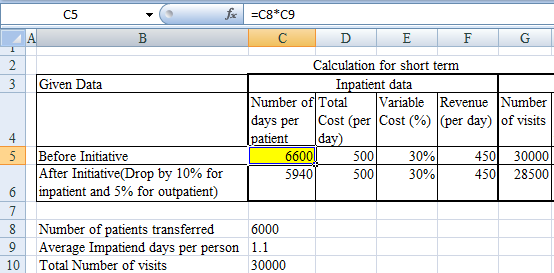Thus, the toal number of days per person is 6600.While the same for OPD is 30,000. Step 2: Calculate the reduction in number of days per patient for inpatient days and OPD.The reduction in inpatient days is made by 10%.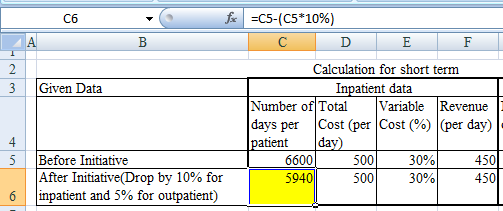Thus, for inpatient utilization the number of days falls to 59410.Calculate 5% deduction for OPD in column G6.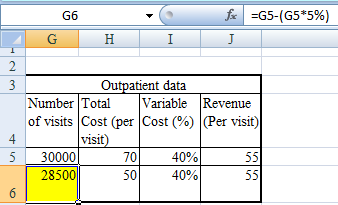Thus the number of days,after considering the initiaive is 28500. The next step is to calculate the fixed cost for the organization.However to calculte the fixed cost, it is pre-requisite to calculate the total cost and variable cost. Step 3 : Calculate total cost by multiplying the total number of days per patient(C5) by the total cost incurred for each (D5) which is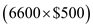.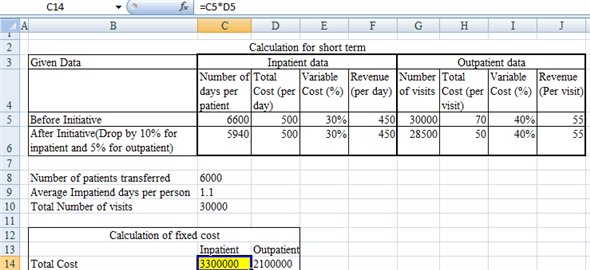Thus, for inpatient utilization the total amount is \$3,300,000.Similarly calculate for OPD by multiplying G5 and H5 which is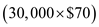.Thus the total cost for OPD is calculated in cell D14 as \$2,100,000. Step 4 : Calculate variable cost by multiplying the total cost in row 14 by the percentage of variable cost.Thus, the variable cost for inpatient utilization is 30% (cell E5).Thus the variable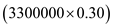.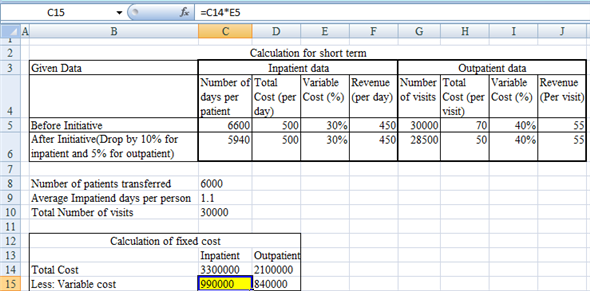Thus, variable cost for inpatient is \$990,000.The percentage of variable cost is 40% for OPD.Thus, similarly variable cost for OPD is calculated by multiplying D14 by I5. The variable cost for OPD is \$840,000. Step 5: Calculate fixed cost by subtracting variable cost (Row 15) from the total cost (Row 14).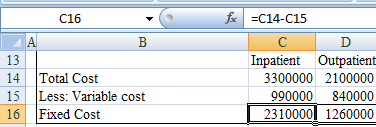Thus, the fixed cost for Inpatient is \$2,310,000 while that for OPD is \$1,260,000. Step 6: Calculate total revenue in by multiplying the revenue per day (F5) by the total number of days per patient (C5). The total revenue for after initiation is calculated by multiplying the data after initiative (Row 6).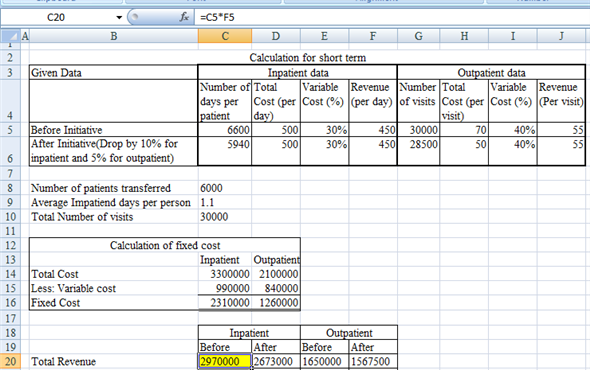Thus, the total revenue before initiation is \$2970000 for inpatient. Similarly, calculate the value for OPD. Step 7: Calculate contribution margin by deducting variable cost (Row 21) from the total revenue (Row 20).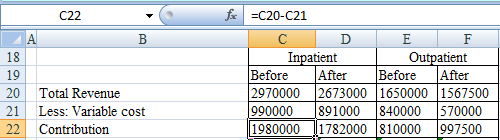Thus, calculate the contribution margin before initiation for inpatient. Copy this value in cell C22 to D22, E22 and F22 to calculate contribution under each alternative. Step 8: Calculation of net profit or loss in Row 24. The loss is calculated by deducting the fixed cost from the amount of contribution. However, it should be noted that the fixed cost will remain same irrespective of initiation of alternative. Thus, the fixed cost is same at \$2,310,000 for inpatient and \$1,260,000 for OPD.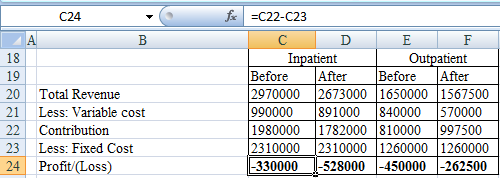The loss is calculated in row 24 by subtracting the fixed cost(Row 23) from contribution margin(Row22). Copy this value in cell C24 to D22, E24 and F24 to calculate profit/loss under each alternative. Step 9: Calculate net loss in Row 29 by summing up the profit or loss under inpatient and OPD, under both situations.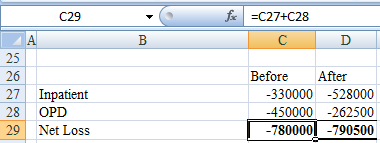As it is observed, that before the initiative is taken net loss is less in comparison to the net loss after the initiative. So firm should not go with this initiative. This is mainly due to the fixed cost. But, if the fixed cost is ignored, that is, in long run, then there is a positive fact that with the increase in net loss and patients by 10% 5 % for in and out patients respectively ,the fixed cost has decline up to 32% in the case of outpatient visits. Decision Rule Hence it can be concluded that this initiative will not be acceptable for short run but will beneficial in the long run.

The Non routine decisions are the decisions which are not taken in normal course of business.They include addition of new services, discontinuing old services, performing tasks such as conducting laboratory tests, engaging into make or buy decisions. So the Non routine decisions play important role in financial position of Healthcare organization as the manager has to take a various decision which affects the working of the organization. They involve t major business decisions which ultimately affect the financial position. Hence, the manager has to be accurate. It is crucial to consider the various costs involved in a proposal. The manager must identify the costs that are capable of affecting investment decisions. Such costs are called relevant cost. The objective of Relevant costing is to show the effect of each alternative on financial result and difference between each alternative result. Hence, relevant cost plays an important role by comparing alternative as it provides the result that may come after selecting particular alternative. It assists the manager in taking efficient decisions by selecting the best (cost effective) alternative. Relevant cost helps the organization whether particular alternative proves to be profitable or loss. They are those costs which are different for different alternatives. There are common costs which occur under all the alternatives. These common costs are ignored while selecting alternatives. It is because they will have same impact on the financial statements. Any costs such as some fixed costs, that will be incurred irrespective of the investment should also be ignored. Thus, costs that are common for all the alternatives should be ignored for simplification of decision process.